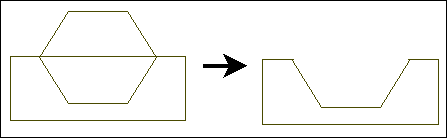# Polygon Subtract

Removes one or more polygons from another polygon.

Point 1: Polygon to be removed (template)

Point 2: Polygon to be subtracted from

The first polygon is removed from the second polygon.To subtract more than one polygon, select the polygons that you want to subtract first. Then choose the Polygon Subtract command and click on the target polygon.

NOTE: To erase the cut lines that remain after this command.